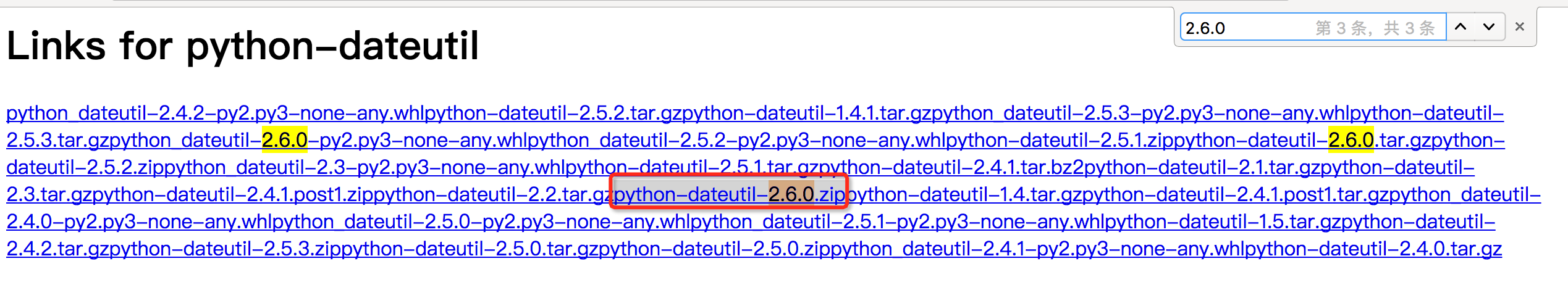#1. 云栖社区>
2. 阿里巴巴大数据 —玩家社区>
3. 博客>
4. 正文

# 背景

PyODPS DataFrame 提供了类似 pandas 的接口，来操作 ODPS 数据，同时也支持在本地使用 pandas，和使用数据库来执行

PyODPS DataFrame 除了支持类似 pandas 的 mapapply 方法，也提供了 MapReduce API 来扩展 pandas 语法以适应大数据环境。

PyODPS 的自定义函数是序列化到 MaxCompute 上执行的，MaxCompute 的 Python 环境只包含了 numpy 这一个第三方包，用户常常问的问题是，如何在自定义函数里使用 pandas、scipy 或者 scikit-learn 这样的包含c代码的库？

PyODPS也需要至少0.7.4版本 。接下来我会详细介绍使用步骤。

# 步骤

## 上传第三方包（只需做一次）

pandas numpy, python-dateutil, pytz, six
scipy numpy
scikit-learn numpy, scipy``add archive python-dateutil.zip;``

pytz 一样，找到 pytz-2017.2.zip。上传不表。

six 找到 six-1.11.0.tar.gz

``add archive pandas.zip;``

python-dateutil python-dateutil-2.6.0.zip python-dateutil.zip
pytz pytz-2017.2.zip pytz.zip
six six-1.11.0.tar.gz six.tar.gz
pandas pandas-0.20.2-cp27-cp27m-manylinux1_x86_64.zip pandas.zip
scipy scipy-0.19.0-cp27-cp27m-manylinux1_x86_64.zip scipy.zip
scikit-learn scikit_learn-0.18.1-cp27-cp27m-manylinux1_x86_64.zip sklearn.zip

## 编写代码验证

``````def test(x):
from sklearn import datasets, svm
from scipy import misc
import numpy as np

assert iris.data.shape == (150, 4)
assert np.array_equal(np.unique(iris.target),  [0, 1, 2])

clf = svm.LinearSVC()
clf.fit(iris.data, iris.target)
pred = clf.predict([[5.0, 3.6, 1.3, 0.25]])
assert pred == 0

assert misc.face().shape is not None

return x``````

``````from odps import options

options.sql.settings = {'odps.isolation.session.enable': True}``````

``````hints = {
'odps.isolation.session.enable': True
}
libraries = ['python-dateutil.zip', 'pytz.zip', 'six.tar.gz', 'pandas.zip', 'scipy.zip', 'sklearn.zip']

iris = o.get_table('pyodps_iris').to_df()

print iris[:1].sepal_length.map(test).execute(hints=hints, libraries=libraries)``````

# PyODPS 相关资源MaxCompute 钉钉群